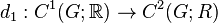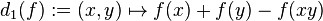Difference between revisions of "Quasimorphism"

WARNING: POTENTIAL TERMINOLOGICAL CONFUSION: Please don't confuse this with quasihomomorphism of groups

Definition

Suppose$G$ is a group. A quasihomomorphism on$G$ is a function$f: G \to \R$ (where$\R$ is the field of real numbers) satisfying the condition that there exists a positive real number$D$ such that for all$x,y \in G$, we have:$|f(xy) - f(x) - f(y)| \le D$

Note that$D$ depends on$f$, but not on the choice of elements of$G$.

The smallest positive real number$D$ that works is called the defect of the quasimorphism$f$. A quasimorphism of defect 0 is the same as a homomorphism to$\R$.

Other names for this concept are quasihomomorphism (not to be confused with a different notion of quasihomomorphism of groups) and pseudocharacter.

Homogenization

A homogeneous quasimorphism is a quasiomorphism that is also a 1-homomorphism of groups, i.e., its restriction to any cyclic subgroup of$G$ is a homomorphism. For any quasimorphism$f$, we can consider its homogenization, defined as$\mu_f := x \mapsto \lim_{n \to \infty} \frac{f(x^n)}{n}$.

Facts

• The collection of all quasimorphisms on a group$G$ is avector space, with the vector space structure being pointwise addition and scalar multiplication of functions. This vector space is denoted$\hat{Q}(G)$. The subspace of homogeneous quasimorphisms is denoted$Q(G)$. The quotient space$\hat{Q}(G)/\operatorname{Hom}(G,\R)$ is a normed vector space with the defect being the norm.

Definition in terms of the language of cohomology

Suppose$G$ is a group. Consider the cochain complex$C^*(G;\R)$ for the trivial group action of$G$ on$\R$. In particular:

•$C^1(G;\R)$ is the additive group of all functions from$G$ to$\R$. In this case,$C^1(G;\R)$ has the structure of a$\R$-vector space.
•$C^2(G;\R)$ is the additive group of all functions from$G \times G$ to$\R$, and also has the structure of a$\R$-vector space.

Consider the coboundary map of the cochain complex:$d_1: C^1(G;\R) \to C^2(G;R)$

given as:$d_1(f) := (x,y) \mapsto f(x) + f(y) - f(xy)$

The standard terminology is as follows:

• The kernel of$d_1$ is the group of 1-cocycles for the trivial group action of$G$ on$\R$, which coincides with the group$\operatorname{Hom}(G;\R)$. Moreover, the group of 1-coboundaries is trivial, so this also coincides with the first cohomology group$H^1(G;\R)$. See first cohomology group for trivial group action is naturally isomorphic to group of homomorphisms.
• The image of$d_1$ is the group of 2-coboundaries for the trivial group action of$G$ on$\R$, and is denoted$B^2(G;\R)$. This, too, is a$\R$-vector space.
• The vector space$\hat{Q}(G)$ that we are interested in is the inverse image under$d_1$ of the vector subspace$B_{bdd}^2(G;\R)$ of$B^2(G;\R)$ comprising the 2-coboundaries that are bounded maps to$\R$.
• By the first isomorphism theorem he quotient space$\hat{Q}(G)/\operatorname{Hom}(G,\R)$ is isomorphic to$B_{bdd}^2(G;\R)$. Moreover, the defect norm on$\hat{Q}(G)/\operatorname{Hom}(G,\R)$ corresponds with the$L^\infty$-norm on this vector space.

Examples

• Any set map from a group to$\R$ with a bounded image is a quasimorphism. In particular, any continuous map from a compact topological group to$\R$ is a quasimorphism. Examples include coordinate projections from compact manifolds embedded in$\R^n$. Note that the homogenization of any such quasimorphism is the zero quasimorphism, so such quasimorphisms are not interesting up to homogenization.
• The rotation number quasimorphism is a homogeneous quasimorphism.